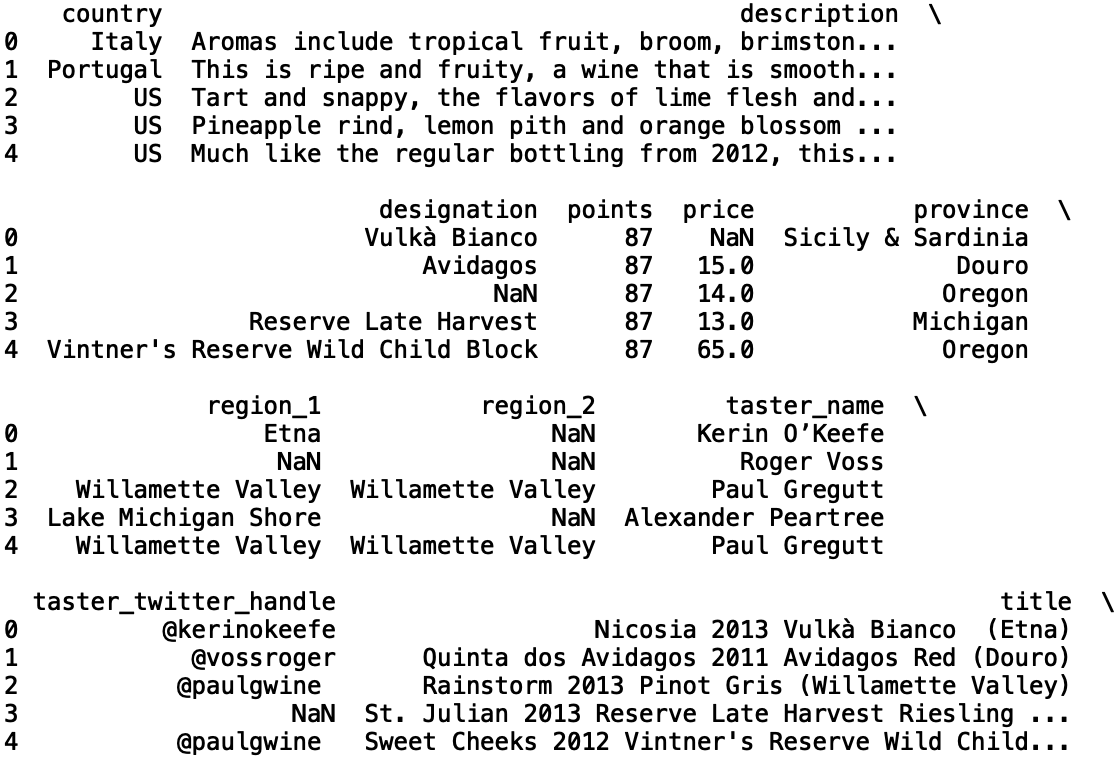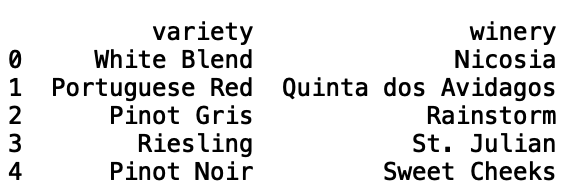# Predicting Missing Values with PythonBuilding Models for Data Imputation. For data scientists, handling missing data is an important part of the data cleaning and model development process. Often times, real data contains multiple sparse fields or fields that are laden with bad values. In this post, we will discuss how to build models that can be used to impute missing or bad values in data.

For data scientists, handling missing data is an important part of the data cleaning and model development process. Often times, real data contains multiple sparse fields or fields that are laden with bad values. In this post, we will discuss how to build models that can be used to impute missing or bad values in data.

Let’s get started!

For our purposes, we will be working with the wines dataset which can be found here.

To start, let’s read the data into a Pandas data frame:

``````import pandas as pd

Next, let’s print the first five rows of data:

``print(df.head())``Let’s take a random sample of 500 records from this data. This will help with speeding up model training and testing, though it can easily be modified by the reader:

``````import pandas as pd
df = pd.read_csv("winemag-data-130k-v2.csv").sample(n=500, random_state = 42)``````

Now, let’s print the info corresponding to our data which will give us an idea of which columns have missing values:

``print(df.info())``

## ML Optimization pt.1 - Gradient Descent with Python

In this article, we explore gradient descent - the grandfather of all optimization techniques and it’s variations. We implement them from scratch with Python.

## Artificial Intelligence (AI) vs Machine Learning vs Deep Learning vs Data Science

Artificial Intelligence (AI) vs Machine Learning vs Deep Learning vs Data Science: Artificial intelligence is a field where set of techniques are used to make computers as smart as humans. Machine learning is a sub domain of artificial intelligence where set of statistical and neural network based algorithms are used for training a computer in doing a smart task. Deep learning is all about neural networks. Deep learning is considered to be a sub field of machine learning. Pytorch and Tensorflow are two popular frameworks that can be used in doing deep learning.

## Data Science With Python Training | Python Data Science Course | Intellipaat

🔵 Intellipaat Data Science with Python course: https://intellipaat.com/python-for-data-science-training/In this Data Science With Python Training video, you...

## Data Science Projects | Data Science | Machine Learning | Python

Practice your skills in Data Science with Python, by learning and then trying all these hands-on, interactive projects, that I have posted for you.

## Data Science Projects | Data Science | Machine Learning | Python

Practice your skills in Data Science with Python, by learning and then trying all these hands-on, interactive projects, that I have posted for you.